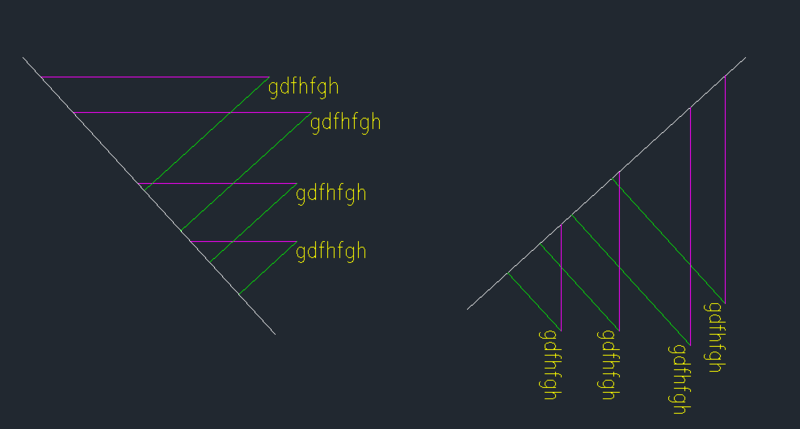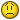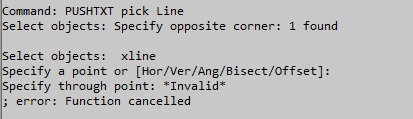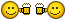Move text (or) Mtext in selected line (or) polyline

Recommended PostsHello Everyone !

The posted code is working fine as per the attached image. They text are move follow with green line direction but I need move text follow with magenta line direction in selected line or Polyline.

Can anyone help me!

Code :

(Defun c:alt  (/ li txts e)
(if
(and (princ "\nSelect Line for Alignment")
(setq li (ssget "_:L" '((0 . "LINE"))))
(princ "\nSelect Texts to Align")
(setq txts (ssget "_:L" '((0 . "TEXT,MTEXT")))))
(repeat (sslength txts)
(vla-move
(setq e (vlax-ename->vla-object
(ssname txts 0)))
(vla-get-insertionpoint e)
(vlax-3d-point
(vlax-curve-getclosestpointto
(ssname li 0)
(vlax-get e 'insertionpoint)))
)
(ssdel (ssname txts 0) txts)
)
)
(princ)
)Share on other sitesAre you happy with move based on angle of  text ? This would use a xline and intersectwith

; By Alan H Oct 2019
; moves text along text angle to a *line
(defun c:pushtxt ( / ss ang obj obj2 obj4 pt1 pt2 pt3 ent)
(setq obj (vlax-ename->vla-object (car (entsel "pick Line "))))
(setq ss (ssget (list (cons 0 "*text"))))
(repeat (setq x (sslength ss))
(setq ent (ssname ss (setq x (- x 1))))
(setq obj2 (vlax-ename->vla-object ent ))
(setq Pt1 (vlax-get Obj2 'Insertionpoint))
(setq ang (vlax-get Obj2 'rotation))
(setq pt3 (polar pt1 ang 10))
(command "xline" pt1 pt3 "")
(setq obj4 (vlax-ename->vla-object (entlast)))
(setq intpt (vlax-invoke obj 'intersectWith obj4 acExtendboth))
(vla-delete obj4)
(command "move" ent "" pt1 intpt)
)
)

(c:pushtxt)

Edited by BIGAL
•1

Share on other sitesAs the OP wants to move to a line or pline I can see a problem arising if the xline cuts the pline more than once, when intpt will become a list min length 6 (2 points), so you would have to find the nearest intersection point.

•1

Share on other sitesNo not workingShare on other sites1 hour ago, Pugazh said:

No not workingHow is it not working?

What error message are you getting?

Are you trying to use this with a polyline or a line?

If with a polyline try it with a line.

Is the visual lisp arx loaded? If not put  (vl-load-com) at the top of the file and try again

•1

Share on other sitesYeah i'm try with line but they text are not move in line.

I got some error See the attached file .Share on other sites10 hours ago, Pugazh said:

Yeah i'm try with line but they text are not move in line.

I got some error See the attached file .

Try this

(defun rh:sammlung_n (o_lst grp / tmp n_lst)
(setq n_lst nil)
(cond ( (and o_lst (= (rem (length o_lst) grp) 0))
(while o_lst
(repeat grp (setq tmp (cons (car o_lst) tmp) o_lst (cdr o_lst)))
(setq n_lst (cons (reverse tmp) n_lst) tmp nil)
);end_while
)
);end_cond
(if n_lst (reverse n_lst))
);end_defun

;; Move Text to Line along text rotation
(defun c:MT2L ( / *error* c_doc c_spc l_obj ent e_lst ss t_obj i_pt t_rot x_obj x_pts s_d x_pt)

(defun *error* ( msg )
(if (not (wcmatch (strcase msg) "*BREAK*,*CANCEL*,*EXIT*")) (princ (strcat "\nOops an Error occurred : " msg)))
(princ)
);end_defun *error*

(setq c_doc (vla-get-activedocument (vlax-get-acad-object))
c_spc (vlax-get-property c_doc (if (= 1 (getvar 'cvport)) 'paperspace 'modelspace))
);end_setq

(while (not l_obj)
(setq ent (car (entsel "\nSelect Line : "))
e_lst (entget ent)
);end_setq
(if (vl-position (cdr (assoc 0 e_lst)) (list "ARC" "LINE" "LWPOLYLINE" "RAY" "SPLINE" "XLINE")) (setq l_obj (vlax-ename->vla-object ent)))
);end_while

(setq ss (ssget '((0 . "*TEXT"))))

(repeat (setq cnt (sslength ss))
(setq t_obj (vlax-ename->vla-object (ssname ss (setq cnt (1- cnt))))
i_pt (vlax-get t_obj 'insertionpoint)
t_rot (vlax-get t_obj 'rotation)
x_obj (vlax-invoke c_spc 'addxline i_pt (polar i_pt t_rot 1.0))
x_pts (rh:sammlung_n (vlax-invoke x_obj 'intersectwith l_obj acextendnone) 3)
s_d 1.0e200
);end_setq

(cond ( (> (length x_pts) 1) (foreach pt x_pts (if (< (distance pt i_pt) s_d) (setq x_pt pt s_d (distance pt i_pt)))))
(t (setq x_pt (car x_pts)))
);end_cond

(vlax-invoke t_obj 'move i_pt x_pt)
(vla-delete x_obj)
);end_repeat
(princ)
);end_defun

It will move the text to the nearest intersection (if more than 1) using the text angle. See attached dwg

•1

Share on other sitesI just tested again and working fine is the angle of the text such that a intersection point is not visually calculated ?

This will force a calculation extending lines replaced in code above

(setq intpt (vlax-invoke obj 'intersectWith obj4 acExtendboth))

Edited by BIGAL
•1

Share on other sitesThank you so much!! yeah both code are now working fineCheers@BIGAL @dlanorh

Join the conversation

You can post now and register later. If you have an account, sign in now to post with your account.
Note: Your post will require moderator approval before it will be visible.Reply to this topic...

×   Pasted as rich text.   Paste as plain text instead

Only 75 emoji are allowed.